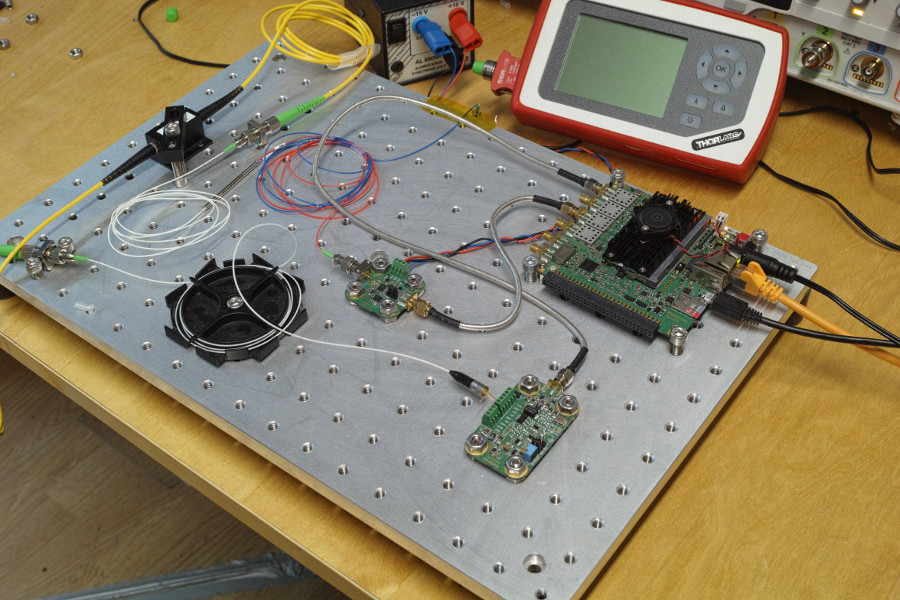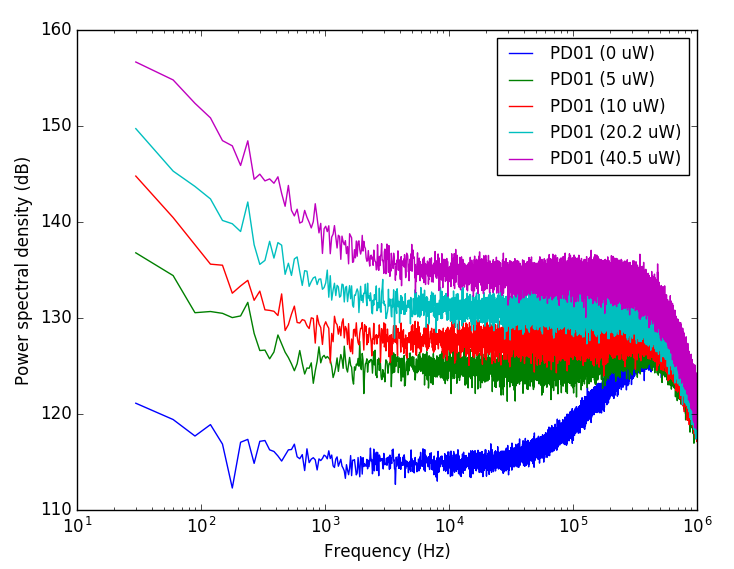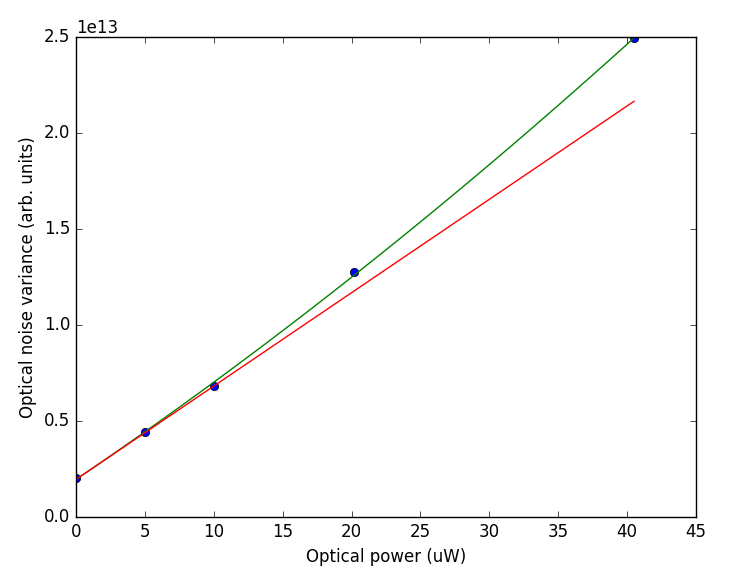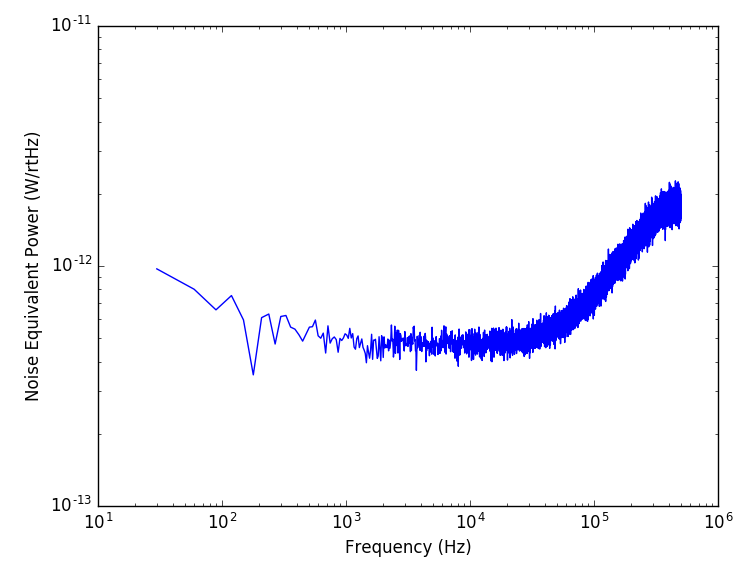# Measuring the Noise Equivalent Power of a photodetector

2018-01-22

Noise Equivalent Power (NEP) is a standard metric for quantifying the sensitivity of photodetectors. In this post we present a method to measure the NEP using optical shot-noise.## Noise Equivalent Power (NEP)

In a photonic system, the optical signal is converted into an electrical signal using a photodetector. Several sources are contributing to the photodetector noise:

• Schottky noise from the photodiode dark current
• Thermal noise
• Amplifier voltage and current noise

Noise can be equivalently given on both sides of the detector either as a voltage noise density vn at the output (in V/√Hz) or as a Noise Equivalent Power (NEP) at the input (in W/√Hz). Both quantities are related by:

$$\mathrm{NEP} = v_{n} / G,$$

where G is the detector gain in V / W.

The NEP defines the incident optical power required to obtain a signal-to-noise ratio (SNR) of 1. For example, when detecting a signal in a Δf = 10 kHz bandwidth with a detector of NEP = 1 pW/√Hz, the optical power required for a SNR of 1 is NEP x (Δf)1/2 = 100 pW.

Usually, the NEP is obtained by measuring G and vn. We show here how to obtain the NEP by relying on the statistical properties of optical shot-noise.

## Measurement setup

The following setup is used for the measurement:A Koheron DRV200 driver controls a DFB laser. The current is set to deliver 2 mW at the laser output. A variable optical attenuator (VOA) is used to control the optical power incident on the Koheron PD01 photodetector. A power meter measures the power delivered to the photodetector. The photodetector output is connected to the input of a Koheron ALPHA250 acquisition board.

On the ALPHA250 acquisition board, we run the adc-dac-dma instrument. This instrument allows us to acquire data buffers up to 8 million samples, resulting in power spectrums spanning from 30 Hz to 125 MHz. A simple Python script acquires a full buffer and performs its Fourier Transform.

## Data analysis

Here are the acquired raw power spectral densities:As expected, the noise increases with the incident optical power. It exhibits 1/f noise followed by a white noise region and then a roll-off induced by the 400 kHz bandwidth of the Koheron PD01 photodetector. The 1/f noise corresponds to the relative intensity noise (RIN) of the DFB laser, whose relative contribution increases with optical power.

It seems that the laser is shot-noise limited at higher frequencies. This is confirmed by plotting the power spectral density at 200 kHz versus the incident optical power:The blue dots are the measured data. The green line is a quadratic fit of the variance:

$$v_{n}^{2} = \mathrm{PSD}_{0} + A P_{\mathrm{opt}} + B P_{\mathrm{opt}}^{2}.$$

The offset is the photodetector noise floor (that is the one we want to convert into a Noise Equivalent Power). The linear term is the optical shot-noise and the quadratic term is the laser RIN. With 40.5 µW of incident power the RIN contributes to about 13 % of the noise at 200 kHz.

The equivalent power of the optical shot-noise is:

$$\mathrm{NEP}_{\mathrm{SN}} = \sqrt{\frac{2 e P_{\mathrm{opt}}}{S}},$$

where e is the elementary charge and S is the photodiode sensitivity (A / W).

Assuming the photodetector response is linear, we have:

$$\frac{\mathrm{NEP}_{\mathrm{SN}}}{\mathrm{NEP}} = \sqrt{\frac{A P_{\mathrm{opt}}}{\mathrm{PSD}_{0}}},$$

therefore

$$\mathrm{NEP} = \sqrt{\frac{2 e \mathrm{PSD}_{0}}{A S}}.$$

Coming back to the initial raw data where PSD0 is the blue curve and using the value of $A$ obtained from the fit and a sensitivity S = 0.9 A/W for the photodiode, we get the Noise Equivalent Power of our photodetector: